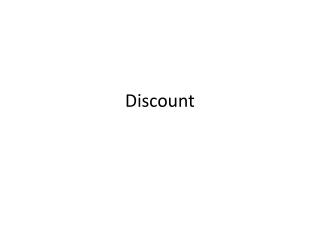DownloadDownload PresentationDiscount

# Discount

Télécharger la présentation## Discount

- - - - - - - - - - - - - - - - - - - - - - - - - - - E N D - - - - - - - - - - - - - - - - - - - - - - - - - - -
##### Presentation Transcript

1. Discount

2. EX1) A stereo’s regular price is \$430. The store is running a sale with a discount of 10%. Find the sale price Method 1 Step 1 Change % to decimal 10% = 0.1 Step 2 Multiply decimal and cost 430 X 0.1 = 43 Round to hundredths if necessary - Step 3 subtract product from original cost 430 43 = \$387

3. EX2) Find the sale price of an item priced \$125 with a discount of 25%. Method 2 Subtract percent of discount from 100% 100% - 25% = 75% Step 1 Change % to decimal Step 2 75% = 0.75 Step 3 Multiply decimal and cost 125 X 0.75 = 93.75 Step 4 Round to hundredths if necessary Sale Price = \$93.75

4. EX3) A cell phone usually sells for \$350, but is on sale for 30% off. What is the total cost of the cell phone with a tax of 7%? 30% = 0.3 350 X 0.3 = 105 Find the discounted price. Step 1 - 350 105 = 245 = \$245 Sale Price Find the tax on the sale price. Step 2 7% = 0.07 X 245 0.7 = 17.15 Step 3 Find final cost + 245 17.15 = 262.15 Step 4 Round to hundredths if necessary Final Cost = \$262.15

5. Assignment Page 279 Problems 1-10 all Skip 7

6. Notes

7. Discount Date _______________

8. EX1) A stereo’s regular price is \$430. The store is running a sale with a discount of 10%. Find the sale price Method 1 Step 1 Change % to decimal Step 2 Multiply decimal and cost Round to hundredths if necessary Step 3 _______ product to original cost

9. EX2) Find the sale price of an item priced \$125 with a discount of 25%. Method 2 _______ percent of discount from 100% Step 1 Change % to decimal Step 2 Step 3 Multiply decimal and cost Step 4 Round to hundredths if necessary

10. EX3) A cell phone usually sells for \$350, but is on sale for 30% off. What is the total cost of the cell phone with a tax of 7%? Find the _________ price. Step 1 Find the ____ on the _____ ____. Step 2 Step 3 Find final cost Step 4 Round to hundredths if necessary